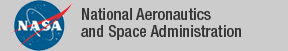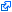### Top File of PVS linear_algebra Library

```
%------------------------------------------------------------------------
%  Top of linear algebra theory   05/12/2013
%------------------------------------------------------------------------
%
%      Index:
%      ------
%
%	sigma_lemmas		-- lemmas about sums
%
%	linear_map		-- definitions about maps
%	sigma_vector		-- summation of vector valued functions
%	linear_map_def		-- definitions and lemmas about linear maps
%
%	vect_of_vect		-- definition of vector of vectors
%	matrices		-- theory about matrices
%	matrix_operator		-- bijection between matrices and linear maps
%	matrix_lemmas		-- basic lemmas about matrices
%	block_matrices		-- theory about block vectors and block matrices
%
% Linear Algebra library
% Heber Herencia-Zapana NIA
% Gilberto Pérez        University of Coruña Spain
% Pablo Ascariz         University of Coruña Spain
% Date: December, 2013
%------------------------------------------------------------------------

top: THEORY

BEGIN

IMPORTING	sigma_lemmas,

linear_map,
sigma_vector,
linear_map_def,

vect_of_vect,
matrices,
matrix_operator,
matrix_lemmas,
block_matrices

END top
```
The tagidentifies links that are outside the NASA domain